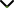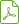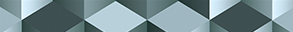# Existence and Asymptotic Behaviour of Solutions of Differential and Integral Equations in Some Function Spaces 2016

Status
Published

Guest Editors

1Rzeszow University of Technology, Rzeszow, Poland

2University of Las Palmas de Gran Canaria, Las Palmas de Gran Canaria, Spain

3Iran University of Science and Technology, Tehran, Iran

# Existence and Asymptotic Behaviour of Solutions of Differential and Integral Equations in Some Function Spaces 2016

## DescriptionIn nonlinear analysis, the solvability of several kinds of operator equations is investigated (differential, integral, functional differential, functional integral, and functional). Usually we look for the solutions of those equations in various function spaces such as Banach function spaces, Sobolev spaces, and Fréchet spaces. It turns out that in order to ensure the solvability of an operator equation in a concrete function space we are forced to impose some assumptions on functions involved in that equation. The method used in the proof of an existence theorem for an operator equation in a given function space also generates various types of constraints on components of that equation.

The aim of this special issue is to investigate the solvability of operator equations in connection with a function space in which our investigations are conducted. Particular emphasis should be laid on various properties of function spaces which are used in the proof of the solvability of considered operator equations. We are also going to study how function spaces, in which operator equations are considered, affect constraints imposed on components of those equations.

The research and review articles are invited which expose the mentioned connections, but this issue is not limited only to papers representing the above described direction and spirit. We invite especially papers concerning miscellaneous aspects of the asymptotic behavior of solutions of differential, integral, and functional equations.

Potential topics include, but are not limited to:

• Operator equations in the space of functions defined, bounded, and continuous on the real half axis
• Operator equations (including partial differential equations) in spaces of functions of several variables
• Solvability of operator equations in the space of functions with increments tempered by a given modulus of continuity
• Operator equations in the space of functions of bounded variation (in classical and generalized sense)
• Operator equations and their solvability in Fréchet spaces
• Solvability of operator equations in other function spaces (Sobolev spaces, absolutely continuous function space, almost periodic function space, etc.)Download Special IssueJournal metrics
See full report
Acceptance rate23%
Submission to final decision72 days
Acceptance to publication20 days
CiteScore2.600
Journal Citation Indicator1.430
Impact Factor1.9Author guidelinesEditorial boardDatabases and indexing

Article of the Year Award: Impactful research contributions of 2022, as selected by our Chief Editors. Discover the winning articles.